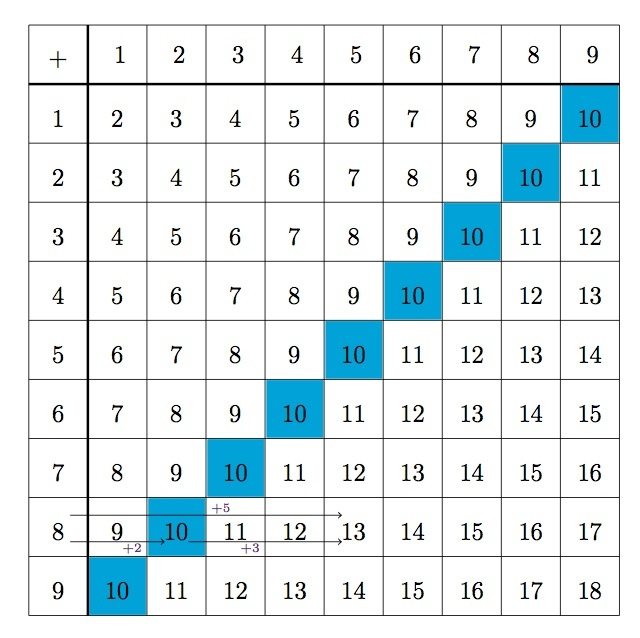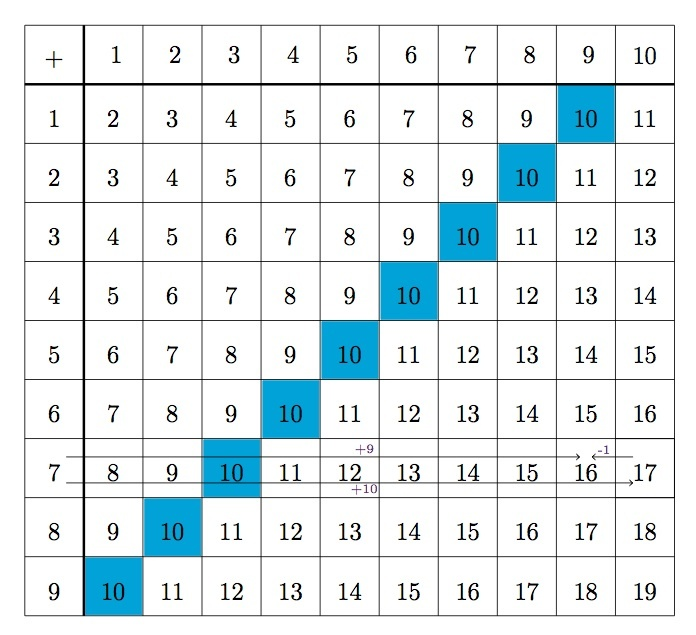# Making a ten

Alignments to Content Standards: 3.OA.D.9

Below is a table showing all the ways to add the numbers from 1 to 9.

1. Each sum which is larger than 10 can be found by first making a 10. For example, to find 8 + 5, we can write
\begin{align} 8+5 &= 8 + (2+3) \\ &= (8+2) + 3 \\ &= 10 + 3 \\ &= 13. \end{align}
Explain why this reasoning works and apply this method to find 7 + 8. How can you visualize these equations using the table?
2. Adding 9 to another single digit number can also be done by first making a 10. For example
\begin{align} 3+9 &= 3 + (10-1) \\ &= (3+10) - 1 \\ &= 13 - 1 \\ &= 12. \end{align}
Explain why this reasoning works and apply this method to find 7+9. How can you visualize these equations using the table?## IM Commentary

The Standards for Mathematical Practice focus on the nature of the learning experiences by attending to the thinking processes and habits of mind that students need to develop in order to attain a deep and flexible understanding of mathematics. Certain tasks lend themselves to the demonstration of specific practices by students. The practices that are observable during exploration of a task depend on how instruction unfolds in the classroom. While it is possible that tasks may be connected to several practices, only one practice connection will be discussed in depth. Possible secondary practice connections may be discussed but not in the same degree of detail.

This task helps illustrate Mathematical Practice 7, Look for and make use of structure. During this activity, third graders are actively connecting the strategy of make-a-ten along with properties of addition and subtraction to solve sums greater than 10.  During this process they are looking at the structure of the base ten system, and composing and decomposing numbers based on this structure.  Students will have the opportunity to think about the meaning and usefulness of composing tens without struggling with the computations which for a third grader will not be challenging.  They are asked to visualize this make-a-ten strategy using the addition table and explain why this reasoning works (MP.3). Through this in-depth exploration of the make-a-ten strategy, students are immersed in pattern-recognition and pattern-generalizing which not only develops a better understanding of our base ten structure but allows them to apply this understanding to solve other problems.

## Solution

1. The first equation comes from writing 5 as 2+3. The reason for this is that in the next step we will group the 2 together with the 8 to make a 10. This second step uses the associative property of addition. In the third step, we add 10 + 3 to find the answer of 13.

In terms of the addition table, we can visualize adding 5 to 8 by starting at the 8 in the column of addends and moving over 5 spaces to the right. Notice that after two steps, we reach 10. Then three more steps puts us at 13. This is shown in the picture below:Applying this method to calculate 7+8 we find

\begin{align} 7+8 &= 7 + (3+5) \\ &= (7+3) + 5 \\ &= 10 + 5 \\ &= 15. \end{align}

2. This method is similar to the making a ten method in part (b). In this case, however, a ten is made by rewriting 9 as 10 - 1. Then the 3 and 10 are grouped together first before performing the subtraction in the final step. This is shown in the picture below:Applying this method to find 7+9 gives

\begin{align} 7+9 &= 7 + (10-1) \\ &= (7+10) - 1 \\ &= 17 - 1 \\ &= 16. \end{align}